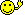CodeGuru Home VC++ / MFC / C++ .NET / C# Visual Basic VB Forums Developer.com

# Thread: Use of Dijkstra's algorithm in PHP

1.Junior MemberJoin Date
Apr 2007
Posts
14

## Use of Dijkstra's algorithm in PHP

Hello everyone! I need some help with Dijkstra's algorithm in PHP!
I have created 3 php files, the one has the map, the other the algorithm and the last one the results.

map.php
HTML Code:
```<html>
<title>Find shortest route!</title>

<script>

function clickMap(classID)
{
if (document.form1.fromClass.value.length == 0)
{
document.form1.fromClass.value = classID;
}
else
{
document.form1.toClass.value = classID;
}
}

</script>
<body>

<img src="teiMap.jpg"  usemap="#routes" border="0">

<MAP name="routes" >
<AREA SHAPE="rect" COORDS="225,50, 300, 100"  href="java script:clickMap('1');" class="mymap" >
<AREA SHAPE="rect" COORDS="400,400,500,500" href="java script:clickMap('3');"  class="mymap" >
</MAP>

<br><br>
<form name="form1" action="calPath.php" method="post">

From :
<input type="text" name="fromClass"/>

<br>
To :
<input type="text" name="toClass"/>
<br>
<input name=b1 type=submit value="Enter!">

</form>

<br></body></html>```
Dijkstra.php
PHP Code:
``` <?php class Dijkstra {     var \$visited = array();     var \$distance = array();     var \$previousNode = array();     var \$startnode =null;     var \$map = array();     var \$infiniteDistance = 0;     var \$numberOfNodes = 0;     var \$bestPath = 0;     var \$matrixWidth = 0;     function Dijkstra(&\$ourMap, \$infiniteDistance) {         \$this -> infiniteDistance = \$infiniteDistance;         \$this -> map = &\$ourMap;         \$this -> numberOfNodes = count(\$ourMap);         \$this -> bestPath = 0;     }     function findShortestPath(\$start,\$to) {         \$this -> startnode = \$start;         for (\$i=0;\$i<\$this -> numberOfNodes;\$i++) {             if (\$i == \$this -> startnode) {                 \$this -> visited[\$i] = true;                 \$this -> distance[\$i] = 0;             } else {                 \$this -> visited[\$i] = false;                 \$this -> distance[\$i] = isset(\$this -> map[\$this -> startnode][\$i])                     ? \$this -> map[\$this -> startnode][\$i]                     : \$this -> infiniteDistance;             }             \$this -> previousNode[\$i] = \$this -> startnode;         }                  \$maxTries = \$this -> numberOfNodes;         \$tries = 0;         while (in_array(false,\$this -> visited,true) && \$tries <= \$maxTries) {                         \$this -> bestPath = \$this->findBestPath(\$this->distance,array_keys(\$this -> visited,false,true));             if(\$to !== null && \$this -> bestPath === \$to) {                 break;             }             \$this -> updateDistanceAndPrevious(\$this -> bestPath);                         \$this -> visited[\$this -> bestPath] = true;             \$tries++;         }     }     function findBestPath(\$ourDistance, \$ourNodesLeft) {         \$bestPath = \$this -> infiniteDistance;         \$bestNode = 0;         for (\$i = 0,\$m=count(\$ourNodesLeft); \$i < \$m; \$i++) {             if(\$ourDistance[\$ourNodesLeft[\$i]] < \$bestPath) {                 \$bestPath = \$ourDistance[\$ourNodesLeft[\$i]];                 \$bestNode = \$ourNodesLeft[\$i];             }         }         return \$bestNode;     }     function updateDistanceAndPrevious(\$obp) {                 for (\$i=0;\$i<\$this -> numberOfNodes;\$i++) {             if(     (isset(\$this->map[\$obp][\$i]))                 &&    (!(\$this->map[\$obp][\$i] == \$this->infiniteDistance) || (\$this->map[\$obp][\$i] == 0 ))                     &&    ((\$this->distance[\$obp] + \$this->map[\$obp][\$i]) < \$this -> distance[\$i])             )                  {                     \$this -> distance[\$i] = \$this -> distance[\$obp] + \$this -> map[\$obp][\$i];                     \$this -> previousNode[\$i] = \$obp;             }         }     }     function printMap(&\$map) {         \$placeholder = ' %' . strlen(\$this -> infiniteDistance) .'d';         \$foo = '';         for(\$i=0,\$im=count(\$map);\$i<\$im;\$i++) {             for (\$k=0,\$m=\$im;\$k<\$m;\$k++) {                 \$foo.= sprintf(\$placeholder, isset(\$map[\$i][\$k]) ? \$map[\$i][\$k] : \$this -> infiniteDistance);             }             \$foo.= "\n";         }         return \$foo;     }     function getResults(\$to) {         \$ourShortestPath = array();         \$foo = '';         for (\$i = 0; \$i < \$this -> numberOfNodes; \$i++) {             if(\$to !== null && \$to !== \$i) {                 continue;             }             \$ourShortestPath[\$i] = array();             \$endNode = null;             \$currNode = \$i;             \$ourShortestPath[\$i][] = \$i;             while (\$endNode === null || \$endNode != \$this -> startnode) {                 \$ourShortestPath[\$i][] = \$this -> previousNode[\$currNode];                 \$endNode = \$this -> previousNode[\$currNode];                 \$currNode = \$this -> previousNode[\$currNode];             }             \$ourShortestPath[\$i] = array_reverse(\$ourShortestPath[\$i]);             if (\$to === null || \$to === \$i) {             if(\$this -> distance[\$i] >= \$this -> infiniteDistance) {                 \$foo .= sprintf("no route from %d to %d. \n",\$this -> startnode,\$i);             } else {                 \$foo .= sprintf(' From %d => to  %d = %d (meters) <br> destinations [%d]: Follow the route to the classes (%s).'."\n" ,                         \$this -> startnode,\$i,\$this -> distance[\$i],                         count(\$ourShortestPath[\$i]),                         implode('-',\$ourShortestPath[\$i]));             }             \$foo .= str_repeat('-',20) . "\n";                 if (\$to === \$i) {                     break;                 }             }         }         return \$foo;     } } // end class ?> ```
calPath.php
PHP Code:
``` <?php include("dijkstra.php"); // I is the infinite distance. define('I',1000); // Size of the matrix \$matrixWidth = 4; // \$points is an array in the following format: (router1,router2,distance-between-them) \$points = array(     array(1,3,7),     array(1,2,1),     array(2,3,1),     array(3,4,2) ); \$ourMap = array(); // Read in the points and push them into the map for (\$i=0,\$m=count(\$points); \$i<\$m; \$i++) {     \$x = \$points[\$i];     \$y = \$points[\$i];     \$c = \$points[\$i];     \$ourMap[\$x][\$y] = \$c;     \$ourMap[\$y][\$x] = \$c; } // ensure that the distance from a node to itself is always zero // Purists may want to edit this bit out. for (\$i=0; \$i < \$matrixWidth; \$i++) {     for (\$k=0; \$k < \$matrixWidth; \$k++) {         if (\$i == \$k) \$ourMap[\$i][\$k] = 0;     } } // initialize the algorithm class \$dijkstra = new Dijkstra(\$ourMap, I,\$matrixWidth); // \$dijkstra->findShortestPath(0,13); to find only path from field 0 to field 13... \$fromClass = \$_POST['fromClass']; \$toClass = \$_POST['toClass']; \$dijkstra->findShortestPath(\$fromClass, \$toClass); // Display the results echo '<pre>'; //echo "the map looks like:\n\n"; //echo \$dijkstra -> printMap(\$ourMap); echo "\n\n the shortest route from class  ".\$fromClass." to ".\$toClass." is  :\n"; echo \$dijkstra -> getResults((int)\$toClass); echo '</pre>'; ?> ```
When I run it I get some warnings and instead of displaying "the fastest route from 1 to 3 is 1-2-3" is says "the fastest route from 1 to 3 is 1-3"!

Could anyone help me??Reply With Quote

2.MemberJoin Date
Dec 2006
Location
Atlanta, GA
Posts
41

## Re: Use of Dijkstra's algorithm in PHP

Maybe it knows something you dont? It seems to me the fastest way from 1 to 3 is 1-3 not 1-2-3, why must you go through 2?

Php is way more advanced then our feeble minds can comprehend, it bends time and space, using infinite probability to get to whatever number it wants to!

So in conclusiong i have no f'ing idea what the dijkstra algorithm is, my highest level of math completed was algebra 2.

Sooorry :*(Reply With Quote

3.Junior MemberJoin Date
Apr 2007
Posts
14

## Re: Use of Dijkstra's algorithm in PHP

that's ok, I made it work, there's a variable somewhere that shouldn't be thereReply With Quote

4.Junior MemberJoin Date
Jan 2008
Posts
1

## Re: Use of Dijkstra's algorithm in PHP

Hi,

Can you tell us what do you change ?

Your dijkstra classes is very interesting for me, can you publish a working version (and of course, can I use it) ?Reply With Quote

5.Junior MemberJoin Date
Jun 2013
Posts
3

## Re: Use of Dijkstra's algorithm in PHP

Hi..,Are you finished this source?Reply With Quote

6.Junior MemberJoin Date
Jun 2013
Posts
3

## Re: Use of Dijkstra's algorithm in PHP

I'm just try to change your array,and the problem solved.

calPath.PHP
PHP Code:
``` <?phpinclude("Dijkstra.php");// I is the infinite distance.define('I',1000);// Size of the matrix\$matrixWidth = 50;// \$points is an array in the following format: (router1,router2,distance-between-them)\$points = array(                    array("1", "2", 6),                    array("1", "6", 8),                    array("1", "3", 4),                    array("2", "4", 5),                    array("2", "1", 6),                    array("2", "3", 2),                    array("3", "1", 4),                    array("3", "2", 2),                    array("3", "5", 2),                    array("4", "2", 5),                    array("4", "5", 4),                    array("5", "3", 2),                    array("5", "4", 4),                    array("5", "6", 8),                    array("6", "1", 8),                    array("6", "5", 8),                                   );\$ourMap = array();// Read in the points and push them into the mapfor (\$i=0,\$m=count(\$points); \$i<\$m; \$i++) {    \$x = \$points[\$i];    \$y = \$points[\$i];    \$c = \$points[\$i];    \$ourMap[\$x][\$y] = \$c;    \$ourMap[\$y][\$x] = \$c;}// ensure that the distance from a node to itself is always zero// Purists may want to edit this bit out.for (\$i=0; \$i < \$matrixWidth; \$i++) {    for (\$k=0; \$k < \$matrixWidth; \$k++) {        if (\$i == \$k) \$ourMap[\$i][\$k] = 0;    }}// initialize the algorithm class\$dijkstra = new Dijkstra(\$ourMap, I,\$matrixWidth);// \$dijkstra->findShortestPath(0,13); to find only path from field 0 to field 13...\$fromClass = \$_POST['fromClass'];\$toClass = \$_POST['toClass'];\$dijkstra->findShortestPath(\$fromClass, \$toClass);// Display the resultsecho '<pre>';//echo "the map looks like:\n\n";//echo \$dijkstra -> printMap(\$ourMap);echo "\n\n the shortest route from class  ".\$fromClass." to ".\$toClass." is  :\n";echo \$dijkstra -> getResults((int)\$toClass);echo '</pre>';?> ```
Last edited by efyeiipe; June 28th, 2013 at 08:33 AM.Reply With Quote

7. ## Re: Use of Dijkstra's algorithm in PHP

Welcome to the forums efyeiipe!Reply With Quote

####Posting Permissions

• You may not post new threads
• You may not post replies
• You may not post attachments
• You may not edit your posts
•